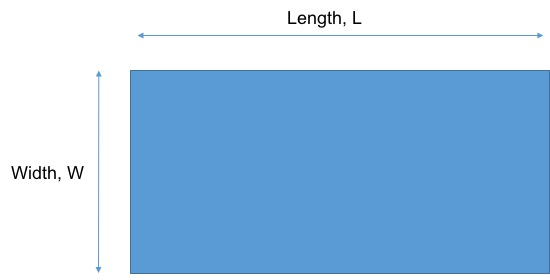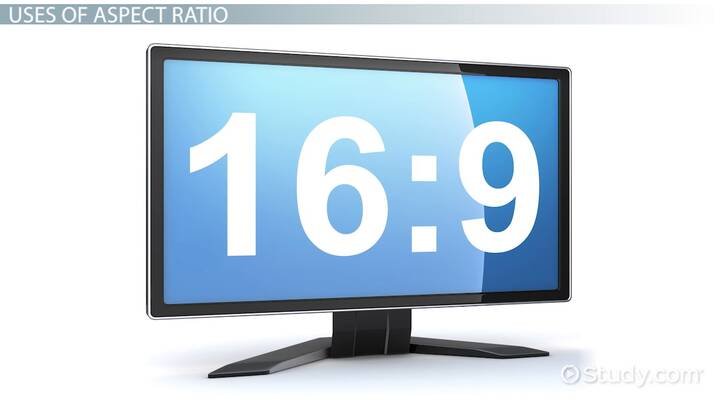# Aspect Ratio: Definition & Calculation

Lesson Transcript
Instructor: Raghav Mahalingam

Raghav has a graduate degree in Engineering and 20 years of professional experience.

This lesson explains the mathematical definition of aspect ratio and a method to calculate the aspect ratio of any given shape. An example calculation is used to compare objects of different aspect ratios. Updated: 08/31/2021

## Aspect Ratio: Definition

Look around you at different objects. Almost all objects around you have dimensions such that one side of the object is typically different (it could be longer or shorter) than an adjacent side. For example, look at your TV screen or your computer screen. Most TV screens have one side larger (or longer) than the other side. An aspect ratio is a mathematical parameter that describes the relationship between the adjacent sides of mechanical objects.

Take a look at this figure appearing here.As you likely know, this shape is called a rectangle, and occurs commonly in objects everywhere around us. Again, for example, look at your computer screen: it's a rectangle. A rectangle has two key defining dimensions, commonly called length (noted here as L, and width, noted here as W).

An aspect ratio can also be described as the ratio between the length (usually the longer dimension) and the width (usually the shorter dimension) of a rectangular shape. Note that since the aspect ratio is defined as the ratio of two lengths, it doesn't have any units and is just a number. An object with a large aspect ratio will appear to be more slender than an object with a small aspect ratio.

Mathematically, aspect ratio (AR) = L / W.

For non-rectangular objects, aspect ratio can be defined using characteristic dimensions of the object. For example, for an ellipse, the aspect ratio can be defined as the ratio of the major to minor axis.An error occurred trying to load this video.

Try refreshing the page, or contact customer support.

Coming up next: What is an Oscilloscope? - Definition & Types

### You're on a roll. Keep up the good work!

Replay
Your next lesson will play in 10 seconds
• 0:04 Aspect Ratio Definition
• 1:38 Example Calculation
• 2:08 Uses of Aspect Ratio
• 2:58 Lesson Summary
Save Save

Want to watch this again later?

Timeline
Autoplay
Autoplay
Speed Speed

## Example Calculation

The length of a widescreen TV is 72 inches and its width is 42 inches. What is the aspect ratio of the screen?

Solution: In this case, L = 72 inches and W = 42 inches

The aspect ratio (AR) = L / W = 72 / 42 = 1.71

To unlock this lesson you must be a Study.com Member.

### Register to view this lesson

Are you a student or a teacher?